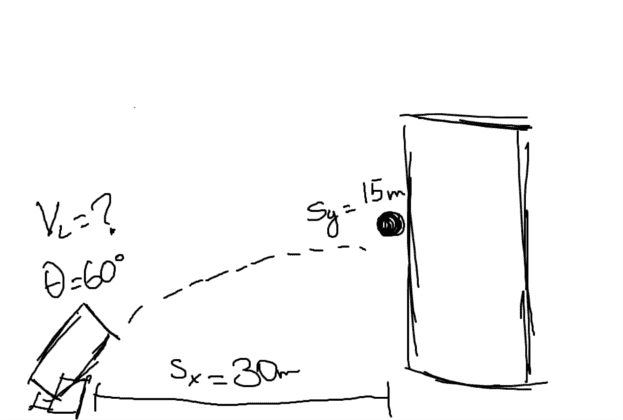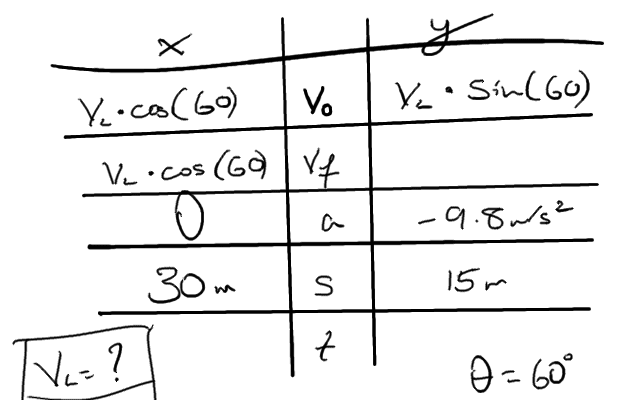# Projectile Motion- 2D Kinematics

## Homework Statement

A mortar elevated to 60 degrees scores a hit 15 m up a building 30 m away. With what speed was the shell fired?

I know I have to find the launch velocity, or VL. Here is a quick sketch i did:s= vot + 1/2at2

v = s/t

## The Attempt at a Solution

I began by setting up a table of known factors:I first set up a distance equation with the x values-

30 = (VL * cos60)t {the second part of the equation presumably cancels out, because acceleration is 0}

Then, I set up the equation using y values-

15 = (VL * sin60)t - 4.9t2

Next I tried solving for time using a different equation using x values:

v = s/t

t = 30/VL * cos60

This being said- do I just plug what I found for t into the x-distance equation? I feel as if I'm not doing the problem correctly because then I would just be squaring the velocity in the x direction and dividing by 30 which leads me back to the original distance. I apologize in advance if the answer is staring at me from right under my nose, I'm not the smartest student. Any and all help is greatly appreciated, thank you so much in advance!

rl.bhat
Homework Helper
15 = (VL * sin60)t - 4.9t^2

Substitute the value of t = 30/(VL*cos60)

15 = VLtan60 - 4.9*30^2/(VLcos60)^2

Now solve for VL.